### Ce cours a été révisé!

Pour une expérience d'apprentissage plus agréable, nous vous recommandons d'étudier la version mobile relogée de ce cours.

Emmenez-moi au cours révisé.

- or -

Continue studying this course

# Mesures de la relation : corrélation et la force de la corrélation

• Notes d'étude
• Révisions du sujet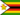Zivanai G. 0 0 A correlation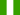LAWRENCE M. 0 0 The correlation coefficient, symbolised by the letter r, extends from -1.00 through 0 to +1.00. A correlation coefficient of -1.00 or +1.00 indicates a perfect correlation. A score between these figures would indicate either a strong correlation or a weak correlation.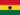Peter Awuni A. 0 0 What is the significance of correlation coefficient?Tamekia Dominquie T. 0 0 correlation coefficient indicates the positive and negative in a relationship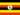Odongo M. 0 0 Psychology -> Measures of relationship: correlation and strength of correlation Measures of relationship: correlation; strength of correlation Relational research attempts to show how two variables change together. The correlation method of investigation is used when a researcher wants to establish the relationship between two variables. The degree of relationship between the two variables is termed the correlation coefficient. A correlation coefficient not only indicates how strongly two variables are related but also the direction, whether it be positive or negative, of the relationship. The correlation coefficient, symbolised by the letter r, extends from -1.00 through 0 to +1.00. A correlation coefficient of -1.00 or +1.00 indicates a perfect correlation. A score between these figures would indicate either a strong correlation or a weak correlation. The closer to zero the figure is, whether it be positive or negative, the weaker the correlation. For example, a correlation of 0.55 indicates a stronger relationship between two variables than a correlation of 0.25 and a correlation of -0.85 indicates an even stronger relationship.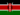Dominic M. 0 0 A good measure of relationship.Dominic M. 0 0 When positive or negative (closer to zero) the weaker the relationship? but are within the perfect range?!Enemchukwu E. 0 0 A correlation is a a way in which two or more variables are related either for positive or negative.Linda T S. 0 0 a correlation of 0.55 indicates a stronger relationship between two variables than a correlation of 0.25 .ABDIKHALIF M Y. 0 0 THIS IS GOOD COURSE